# Comparison of AMP_SF Correction Methods

## Introduction

In an earlier memo, I described an algorithm for correcting the telemetered values of the crossed-grid charge detector amplifier scale factor (AMP_SF) on an event-by-event basis. The algorithm relied on the expectation that the sum of the six taps that are telemetered for event positions should be roughly proportional to the PHA to within the scale factor and that the correct scale factor should change with event amplitude as measured by the PHA. Steve Murray has provided an alternative method for doing this correction which has some differences. Steve's method is described in the following e-mail excerpt:
```
/*AMP_SF Test and Fix */
sum_amps = 0.5*( evt->au1 + evt->au2 + evt->au3 + evt->av1 +
evt->av2 + evt->av3 )/sfparm->amp_gain;
if( evt->pha < 255 ) {
diff1 = fabs(evt->pha - sum_amps);
diff2 = fabs(evt->pha - 2.0*sum_amps);
diff3 = fabs(evt->pha - 4.0*sum_amps);
if( (diff1 <= diff2) && (diff1 <= diff3) &&
(diff1 < sfparm->pha_tol1) ) {
sf = 1;
} else if( (diff2 <= diff1) && (diff2 <= diff3) &&
(diff2 < sfparm->pha_tol2) ) {
sf = 2;
} else if( (diff3 <= diff1) && (diff3 <= diff2) &&
(diff3 < sfparm->pha_tol3) ) {
sf = 3;
} else {
sf = 0;
}
if( sf == 0 ) {
sfparm->ampsf_cnt++;
}
} else {
sf = 3;
}
if ( sf != evt->amp_sf ) {
sfparm->fixampsf_cnt++;
evt->amp_sf = sf;
}
.
.
.
The key parameters are the gains used to convert summed amplifiers to PHA
(74.0 for HRC-I and 52.9 for HRC-S), and the tolerances for agreement (20 for
HRC-I and 50 for HRC-S - this is a poor guess).

```

In an effort to compare these two techniques I have implemented both correction methods as routines that can operate on the CXCDS HRC level 0 events list. I have slightly modified Steve's method in that rather than setting the scale factor to zero if the event is not in one of the normal three ranges, my routine leaves AMP_SF unchanged. In addition I do not set any status bits. The figure below is a graphical representation of this identification technique.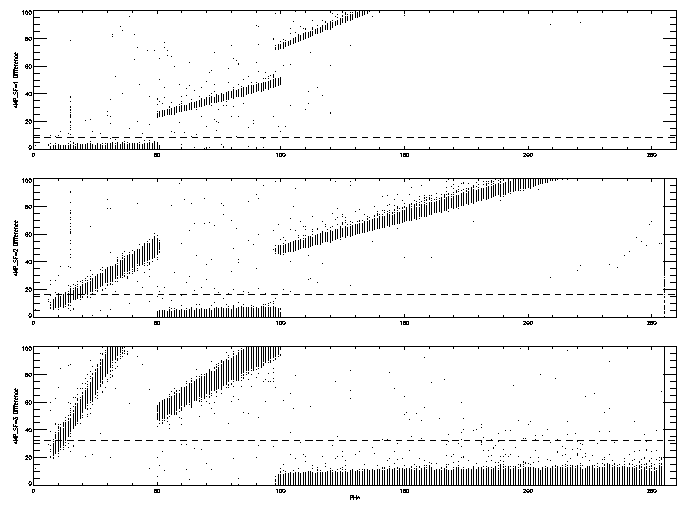(postsctipt - 4.2MB)

The absolute value of the difference of the scaled sum of the six taps (normalized in gain relative to the PHA scale) is plotted versus PHA for each of the possible scale factors. The horizontal dashed lines are the tolerance band. In this case I used a different "tolerance" on each of the scale ranges (8, 16, and 32 for AMP_SF values of 1, 2, and 3 respectively) rather than the fixed value of 20. This approach can be compared to figure 1 from my earlier memo, where a line forms a boundary between regions of different AMP_SF.

Applying this method to data from the HRC-S is somewhat trickier as the figure below demonstrates. This figure is similar to the one above except that the normalization on the sum of the six taps is different (105.8 instead of 148.0) and the tolerance is 50 for AMP_SF=1 and 2 and 100 for AMP_SF=3.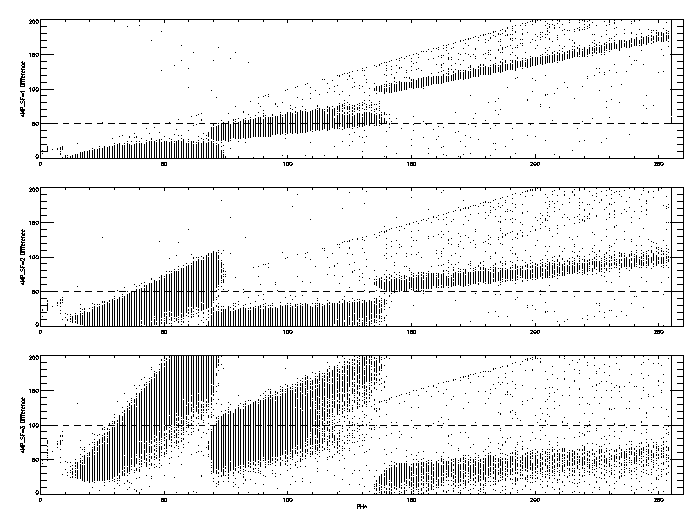(postscript - 5.0MB)

The potential for mis-identification of the scale factor by this method can be seen in the events with PHA values less than 50. Around these PHA value we expect the events to have AMP_SF values of 1; however, many of the events have difference values near zero when AMP_SF=2. A similar problem exists in the algorithm that I presented in the earlier memo, where a constant slope can clip the lower end of the range of the sum of the tap values at a given PHA (see figure 2 from my earlier memo).

## HRC-I Comparison Results

I performed a comparison of the two methods of correcting the AMP_SF values using a sub-set of events from the 20 ks observation of AR-Lac (ObsID 1385). I selected events from coarse positions that contained events from AR-Lac (CRSU=28-30, CRSV=29-30) and used both methods to determine a corrected AMP_SF. The following table gives the number of events corrected by the two methods. In what follows Method 1 is my implementation of Steve's algorithm while Method 2 is the algorithm from my earlier memo.

 Total number of events 135074 Corrected by Method 1 324 Corrected by Method 2 437 Corrected by Method 1 but not by Method 2 5 Corrected by Method 2 but not by Method 1 118 Corrected by Method 1 and by Method 2 but different values 1

A graphical comparison is shown in the following figure, plotting the "scaled" sum of the six tap readouts (SUMAMPS×2AMP_SF-1/148) versus the event PHA. In all three panes the telemetered values for the amplifier scale are used rather than the corrected ones. Events that receive a correction are shown as "+" symbols. In the top pane the "+" indicates which events were corrected via Method 1; in the middle pane the "+" indicates those events corrected by Method 2; and in the bottom pane the "+" indicates events where the two correction methods yield different results (the 124 events in the last three row of the table above).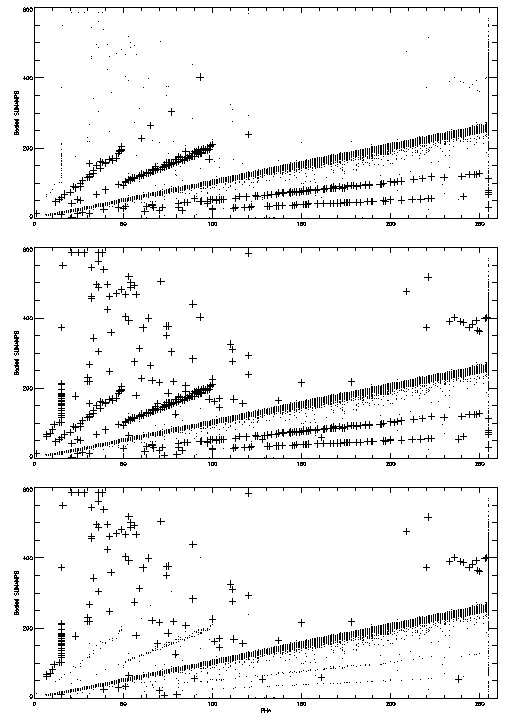(postscript - 5.9MB)

For the most part the two methods apply corrections to the branches at 1/4×, 1/2×, 2×, and 4× the expected scale factor. As expected, the events that Method 1 does not correct lie outside bands centered on these branches.

An additional comparison can be made by plotting the scaled sum of the six taps using the corrected scale factor versus PHA; this is done in the figure below. The pane on the left was corrected using Method 1 while the pane on the right used Method 2. In both panes the red "+" signs indicate the events for which the two methods yielded different results.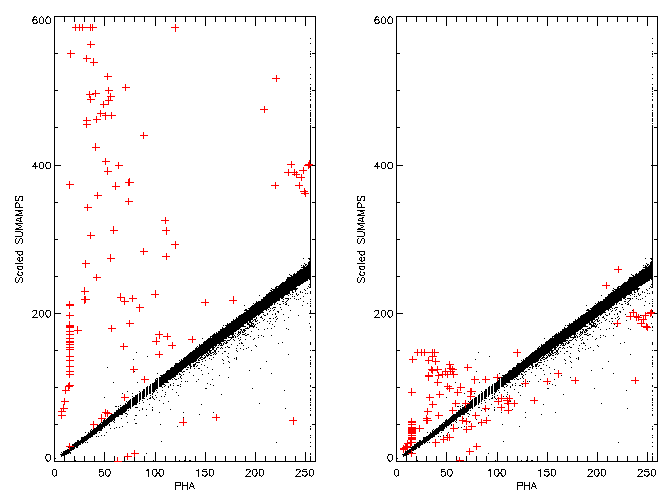(postscript - 3.8MB)

For the HRC-I both methods perform equally well in correcting the AMP_SF values.

## HRC-S Comparison Results

I performed a similar comparison for the HRC-S using the zero-order region of a recent HRC-S/LETG observation. I selected all events from coarse positions that contained the zero-order image (CRSU=7-9, CRSV=97-100). A tabulation of the total number of events and the numbers corrected by the two methods is given below.

 Total number of events 130416 Corrected by Method 1 6927 Corrected by Method 2 8265 Corrected by Method 1 but not by Method 2 14 Corrected by Method 2 but not by Method 1 1352 Corrected by Method 1 and by Method 2 but different values 6

Plots similar to those for the HRC-I comparison are displayed below. First we plot the scaled sum of the six taps, using the uncorrected AMP_SF values as a function of PHA. The three panes once again identify events corrected by Method 1 (top), those corrected by Method 2 (center), and those where the two methods gave different results (bottom).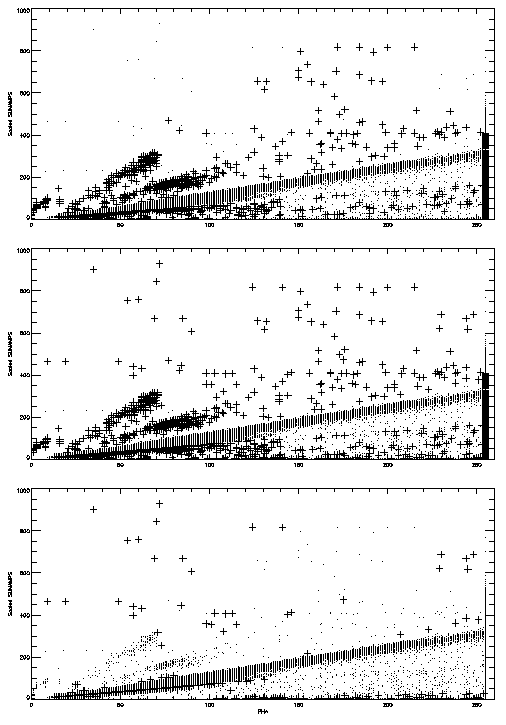(postscript - 6.2MB)

Below are plots of the scaled sum of the six taps versus PHA after AMP_SF correction (Method 1 - left, Method 2 - right). The red "+" symbols identify events where the two method produce different results.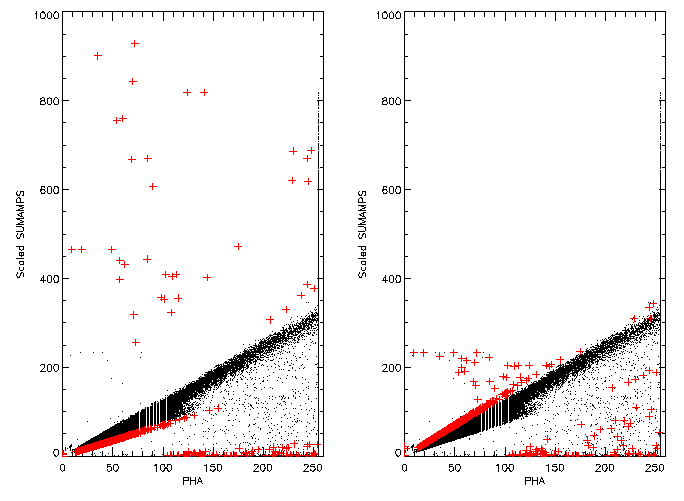(postscript - 3.8MB)

Once again the results of the two methods are comparable. However, both methods change the AMP_SF value to 2 for many events in the PHA region where we would normally expect a value of 1; Method 2, using a SUMAMPS to PHA ratio of 38, seems to do this for a few more events than Method 1. This mis-assignment is caused by the broad distribution of SUMAMPS at a fixed PHA for low PHA values and is intrinsic to both methods.

## Conclusions

The two methods that have been developed for correcting the AMP_SF values produce similar results. Since both methods relied on an expected linear relationship between the scaled sum of the six taps and the PHA, this was to be expected. While both methods produce excellent results when processing HRC-I data, both methods have a similar problem when correcting the HRC-S data due to the looser correlation between the sum of the six tap values and the PHA observed on the HRC-S. A side-by-side comparison of the results of the two methods does not suggest a preference of one over the other. Other factors (ease of implementation within the existing structure, processing speed, "taste") may play a deciding role in selecting between them for inclusion in standard processing.

Mike Juda